RD Sharma 2013 Solutions for Class 6 Math Chapter 4 Operations On Whole Numbers are provided here with simple step-by-step explanations. These solutions for Operations On Whole Numbers are extremely popular among class 6 students for Math Operations On Whole Numbers Solutions come handy for quickly completing your homework and preparing for exams. All questions and answers from the RD Sharma 2013 Book of class 6 Math Chapter 4 are provided here for you for free. You will also love the ad-free experience on Meritnation’s RD Sharma 2013 Solutions. All RD Sharma 2013 Solutions for class 6 Math are prepared by experts and are 100% accurate.

#### Question 1:

Fill in the blanks to make each of the following a true statement:

(i) 785 × 0=....

(ii) 4567 × 1=...

(iii) 475 × 129=129 × ....

(iv) .... × 8975=8975 × 1243

(v) 10 × 100 × ...=10000

(vi) 27 × 18=27 × 9+27 × ...+27 × 5

(vii) 12 × 45=12 × 50 − 12 × ...

(viii) 78 × 89=78 × 100 − 78...+78 × 5

(ix) 66 × 85=66 × 90 − 66 × ... − 66

(x) 49 × 66+49 × 34=49 × (...+...)

#### Answer:

(i) 785 × 0 = 0

(ii) 4567 × 1 = 4567 (Multiplicative identity)

(iii) 475 × 129 = 129 × 475 (Commutativity)

(iv) 1243 × 8975 = 8975 × 1243 (Commutativity)

(v) 10 × 100 × 10 = 10000

(vi) 27 × 18 = 27 × 9 + 27 × + 27 × 5

(vii) 12 × 45 = 12 × 50 − 12 ×  5

(viii) 78 × 89 = 78 × 100 − 78 × 16 + 78 × 5

(ix) 66 × 85 = 66 × 90 − 66 × 4 − 66

(x) 49 × 66 + 49 × 34 = 49 × (66 + 34)

#### Question 2:

Determine each of the following products by suitable rearrangements:

(i) 2 × 1497 × 50

(ii) 4 × 358 × 25

(iii) 495 × 625 × 16

(iv) 625 × 20 × 8 × 50

#### Answer:

(i) 2 × 1497 × 50
= (2 × 50) × 1497 = 100 × 1497 = 149700

(ii) 4 × 358 × 25
= (4 × 25) × 358 = 100 × 358 = 35800

(iii) 495 × 625 × 16
= (625 × 16) × 495 = 10000 × 495 = 4950000

(iv) 625 × 20 × 8 × 50
= (625 × 8) × (20 × 50) = 5000 × 1000 = 5000000

#### Question 3:

Using distributivity of multiplication over addition of whole numbers, find each of the following products:

(i) 736 × 103
(ii) 258 × 1008
(iii) 258 × 1008

#### Answer:

(i) 736 × 103
= 736 × (100 + 3)
{Using distributivity of multiplication over addition of whole numbers}
= (736 × 100)  + (736 × 3)
= 73600 + 2208 = 75808

(ii) 258 × 1008
= 258 × (1000 + 8)
{Using distributivity of multiplication over addition of whole numbers}
= (258 × 1000)  + (258 × 8)
= 258000 + 2064 = 260064

(iii) 258 × 1008
= 258 × (1000 + 8)
{Using distributivity of multiplication over addition of whole numbers}
= (258 × 1000)  + (258 × 8)
= 258000 + 2064 = 260064

#### Question 4:

Find each of the following products.

(i) 736 × 93
(ii) 816 × 745
(iii) 2032 × 613

#### Answer:

(i) 736 × 93
∵ 93 = (100 − 7)
∴ 736 × (100 − 7)
= (736 × 100) − ( 736 × 7)
(Using distributivity of multiplication over subtraction of whole numbers)
= 73600 − 5152 = 68448

(ii) 816 × 745
∵ 745 = (750 − 5)
∴ 816 × (750 − 5)
= (816 × 750) − ( 816 × 5)
(Using distributivity of multiplication over subtraction of whole numbers)
= 612000 − 4080 = 607920

(iii) 2032 × 613
∵ 613 = (600 +13)
∴ 2032 × (600 + 13)
= (2032 × 600) + ( 2032 × 13)
= 1219200 + 26416 = 1245616

#### Question 5:

Find the values of each of the following using properties:

(i) 493 × 8 + 493 × 2

(ii) 24579 × 93 + 7 × 24579

(iii) 1568 × 184 − 1568 × 84

(iv) 15625 × 15625 − 15625 × 5625

#### Answer:

(i) 493 × 8 + 493 × 2
= 493 × (8 + 2)
(Using distributivity of multiplication over addition of whole numbers)
= 493 × 10 = 4930

(ii) 24579 × 93 + 7 × 24579
= 24579 × (93 + 7)
(Using distributivity of multiplication over addition of whole numbers)
= 24579 × 100 = 2457900

(iii) 1568 × 184 − 1568 × 84
= 1568 × (184 − 84)
(Using distributivity of multiplication over subtraction of whole numbers)
= 1568 × 100 = 156800

(iv) 15625 × 15625 − 15625 × 5625
= 15625 × (15625 − 5625)
(Using distributivity of multiplication over subtraction of whole numbers)
= 15625 × 10000 = 156250000

#### Question 6:

Determine the product of:

(i) the greatest number of four digits and the smallest number of three digits.
(ii) the greatest number of five digits and the greatest number of three digits.

#### Answer:

(i) The largest four−digit number = 9999
The smallest three−digit number = 100
∴ Product of the smallest three−digit number and the largest four−digit number  = 9999 × 100 = 999900

(ii)
The largest five-digit number = 9999
The largest number of three digits = 999
∴ Product of the largest three-digit number and the largest five−digit number = 9999 × 999
= 9999 × (1000 − 1)
= (9999 × 1000) − (9999 × 1)
= 9999000 − 9999 = 9989001

#### Question 7:

In each of the following, fill in the blanks, so that the statement is true:

(i) (500 + 7) (300 − 1) = 299 × ...

(ii) 888 + 777 + 555 = 111 × ....

(iii) 75 × 425 = (70 + 5) (... + 85)

(iv) 89 × (100 − 2) × 98 × (100 − ....)

(v) (15 + 5) (15 − 5) = 225 − ....

(vi) 9 × (10000 + ...) = 98766

#### Answer:

(i) (500 + 7) (300 − 1) = 507 × 299 = 299 × 507 (Commutativity)

(ii) 888 + 777 + 555 = 111 (8 + 7 + 5) = 111 × 20  (Distributivity)

(iii) 75 × 425 = (70 + 5) × 425 = (70 + 5) (340 + 85)

(iv) 89 × (100 − 2) = 89 × 98 = 98 × 89 = 98 × (100 − 11)  (Commutativity)

(v) (15 + 5) (15 − 5) =  20 × 10 = 200 = 225 − 25

(vi) 9 × (10000 + 974) = 98766

#### Question 8:

A dealer purchased 125 colour television sets. If the cost of each set is Rs 19820, determine the cost of all sets together.

#### Answer:

Cost of 1 colour television set =  Rs 19820
∴ Cost of  125 colour television sets = Rs (19820 × 125)
= Rs 19820 × (100 + 25)
= Rs (19820 × 100) + (19820 × 25)
= Rs 1982000 + 495500
= Rs 2477500

#### Question 9:

The annual fee charged from a student of class VI in a school is Rs 8880. If there are, in all, 235 students in class VI, find the total collection.

#### Answer:

Fees charged from 1 student = Rs 8880
∴ Fees charged from 235 students = Rs 8880 × 235 = 2086800
Thus, the total collection from class VI students is Rs 2086800.

#### Question 10:

A group housing society constructed 350 flats. If the cost of construction for each flat is Rs 993570, what is the total cost of construction of all the flats.

#### Answer:

Cost of construction of 1 flat = Rs 993,570
Total number of flats constructed = 350
Total cost of construction of 350 flats = Rs (993,570 × 350) = Rs 347,749,500

#### Question 11:

The product of two whole numbers is zero. What do you conclude?

#### Answer:

If the product of two whole numbers is zero, then it means that either one of them is zero or both of them are zero.

#### Question 12:

What are the whole numbers which when multiplied with itself gives the same number?

#### Answer:

There are two numbers which when multiplied with themselves give the same numbers.

(i) 0 × 0 = 0
(ii) 1 × 1 = 1

#### Question 13:

In a large housing complex, there are 15 small buildings and 22 large building. Each of the large buildings has 10 floor with 2 apartments on each floor. Each of the small buildings has 12 floors with 3 apartments on each floor. How many apartments are there in all.

#### Answer:

Number of large buildings = 22
Number of small buildings = 15
Number of floors in 1 large building = 10
Number of apartments on 1 floor = 2
∴ Total apartments in 1 large building = 10 × 2 = 20
Similarly,
​Total apartments in 1 small building = 12 × 3 = 36

∴​ Total apartments in the entire housing complex = (22 × 20) + (15 × 36)
= 440 + 540
= 980

#### Question 1:

Does there exist a whole number a such that a $÷$ a = a?

#### Answer:

Yes, there exists a whole number a such that a $÷$ a = a.
The whole number is 1 such that .

#### Question 2:

Find the value of:

(i) 23457 $÷$ 1
(ii) 0 $÷$ 97
(iii) 476 + (840 $÷$ 84)
(iv) 964 − (425 $÷$ 425)
(v) (2758 $÷$ 2758) − (2758 $÷$ 2758)
(vi) 72450 $÷$ (583 − 58)

#### Answer:

(i) 23457 $÷$ 1 = 23457
(ii) 0 $÷$ 97 = 0
(iii) 476 + (840 ÷ 84) = 476 + 10 = 486
(iv) 964 − (425 ÷ 425) = 964 − 1 = 963
(v) (2758 ÷ 2758) − (2758 ÷ 2758) = 1 − 1 = 0
(vi) 72450 ÷ (583 − 58) = 72450 ÷ 525 = 138

#### Question 3:

Which of the following statements are true:

(i) 10 $÷$ (5 × 2) = (10 $÷$ 5) × (10 $÷$ 2)
(ii) (35 − 14) $÷$ 7 = 35 $÷$ 7 − 14 $÷$ 7
(iii) 35 − 14 $÷$ 7 = 35 $÷$ 7 − 14 $÷$ 7
(iv) (20 − 5) $÷$ 5 = 20 $÷$ 5 − 5
(v) 12 × (14 $÷$ 7) = (12 × 14) $÷$ (12 × 7)
(vi) (20 $÷$ 5) $÷$ 2 = (20 $÷$ 2) $÷$ 5

#### Answer:

(i) False
LHS: 10 ÷ (5 × 2)
= 10 ÷ 10
= 1
RHS: (10 ÷ 5) × (10 ÷ 2)
= 2 × 5
= 10

(ii) True
LHS: (35 − 14) ÷ 7
= 21 ÷ 7
= 3
RHS: 35 ÷ 7 − 14 ÷ 7
= 5 − 2
= 3

(iii) False
LHS: 35 − 14 ÷ 7
= 35 − 2
= 33
RHS: 35 ÷ 7 − 14 ÷ 7
= 5 − 2
= 3

(iv) False
LHS: (20 − 5) ÷ 5
= 15 ÷ 5
= 3
RHS: 20 ÷ 5 − 5
= 4 − 5
= −1

(v) False
LHS: 12 × (14 ÷ 7)
= 12 × 2
= 24
RHS: (12 × 14) ÷ (12 × 7)
= 168 ÷ 84
= 2

(vi) True
LHS: (20 ÷ 5) ÷ 2
= 4 ÷ 2
= 2
RHS: (20 ÷ 2) ÷ 5
= 10 ÷ 5
= 2

#### Question 4:

Divide and check the quotient and remainder:

(i) 7772 $÷$ 58
(ii) 6906 $÷$ 35
(iii) 16135 $÷$ 875
(iv) 16025 $÷$ 1000

#### Answer:

(i) 7777 ÷ 58 = 134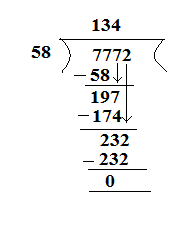Verification: [Dividend  = Divisor × Quotient + Remainder]
7772 = 58 × 134 + 0
7772 = 7772
LHS = RHS

(ii)  6906 ÷ 35 gives quotient = 197 and remainder = 11.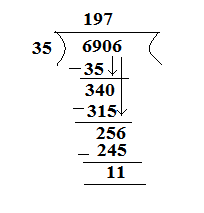Verification: [Dividend  = Divisor × Quotient + Remainder]
6906  =  35 × 197 + 11
6906 = 6895 + 11
6906 = 6906
LHS = RHS

(iii) 16135 ÷ 875 gives quotient = 18 and remainder = 385.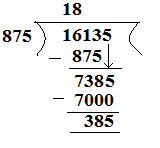Verification: [Dividend  = Divisor × Quotient + Remainder]
16135 = 875 × 18 + 385
16135 = 15750 + 385
`     16135 = 16135
LHS = RHS

(iv) 16025 ÷ 1000 gives quotient = 16 and remainder = 25.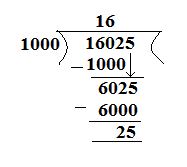Verification: [Dividend  = Divisor × Quotient + Remainder]
16025 = 1000 × 16 + 25
16025 = 16000 + 25
16025 = 16025
LHS = RHS

#### Question 5:

Find a number which when divided by 35 gives the quotient 20 and remainder 18.

#### Answer:

Dividend = Divisor × Quotient + Remainder
Dividend = 35 × 20 + 18
= 700 +18
= 718

#### Question 6:

Find the number which when divided by 58 gives a quotient 40 and remainder 31.

#### Answer:

Dividend =  Divisor × Quotient + Remainder
Dividend = 58 × 40 + 31
= 2320 + 31
= 2351

#### Question 7:

The product of two numbers is 504347. If one of the numbers is 1591, find the other.

#### Answer:

Product of two numbers = 504347
One of the two numbers = 1591
Let the number be A.
∴ A × 1591 = 504347

A = $\frac{504347}{1591}$ = 317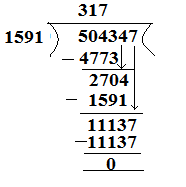#### Question 8:

On dividing 59761 by a certain number, the quotient is 189 and the remainder is 37. Find the divisor.

#### Answer:

Dividend = 59761
Quotient = 189
Remainder = 37
Divisor = A
Now, Dividend = Divisor × Quotient + Remainder
59761 = A × 189 + 37
59761 − 37 = A × 189
59724 = A × 18

A = $\frac{59724}{189}$ = 316

#### Question 9:

On dividing 55390 by 299, the remainder is 75. Find the quotient.

#### Answer:

Dividend = 55390
Divisor = 299
Remainder = 75
Quotient = A
Dividend = Divisor × Quotient + Remainder
55390 = 299 × A + 75
55390 - 75 = A × 299
55315 = A × 299

A = $\frac{55315}{299}$ = 185

#### Question 1:

Without drawing a diagram, find

(i) 10th square number
(ii) 6th triangular number.

#### Answer:

(i) 10th square number: A square number can easily be remembered by the following rule:
nth square number = n2
∴ 10th square number = 102 = 100

(ii) 6th triangular number: A triangular number can easily be remembered by the following rule:
nth triangular number = $\frac{n×\left(n+1\right)}{2}$
∴ 6th triangular number = $\frac{6×\left(6+1\right)}{2}$ = 21

#### Question 2:

(i) Can a rectangular number also be a square number?
(ii) Can a triangular number also be a square number?

#### Answer:

(i) Yes, a rectangular number can also be a square number; for example, 16 is a square number and also a rectangular number.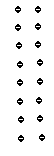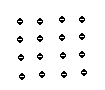(ii) Yes, there exists only one triangular number that is both a triangular number and a square number, and that number is 1.

#### Question 3:

Write the first four products of two numbers with difference 4 starting from in the following order:

1,2,3,4,5,6.....

#### Answer:

1 $×$ 5 = 5    (5 − 1 = 4)
2 $×$ 6 = 12  (6 − 2  = 4)
3 $×$ 7 = 21  (7 − 3 = 4)
4 $×$ 8 = 32  (8 − 4 = 4)

#### Question 4:

Observe the pattern in the following and fill in the blanks:

9 × 9 + 7              = 88
98 × 9 + 6            = 888
987 × 9 + 5          = 8888
9876 × 9 + 4        = ...............
98765 × 9 + 3      = ...............
987654 × 9 + 2    = ...............
9876543 × 9 + 1  = ...............

#### Answer:

9 × 9 + 7              = 88
98 × 9 + 6            = 888
987 × 9 + 5          = 8888
9876 × 9 + 4        = 88888
98765 × 9 + 3      = 888888
987654 × 9 + 2    = 8888888
9876543 × 9 + 1  = 88888888

#### Question 5:

Observe the following pattern and extend it to three more steps:

6 × 2 −  5        = 7
7 × 3 − 12       = 9
8 × 4 − 21       = 11
9 × 5 − 32       = 13
.... × .... − ..... = ......
.... × .... − ..... = ......
.... × .... − ..... = ......

#### Answer:

6 × 2 −  5        = 7
7 × 3 − 12       = 9
8 × 4 − 21       = 11
9 × 5 − 32       = 13
10 × 6 − 45       = 15
11 × 7 − 60       = 17
12 × 8 − 77       = 19

#### Question 6:

Study the following pattern:

1 + 3                       = 2 × 2
1 + 3 + 5                = 3 × 3
1 + 3 + 5 + 7         = 4 × 4
1 + 3 + 5 + 7 + 9   = 5 × 5

By observing the above pattern, find

(i) 1 + 3 + 5 + 7 + 9 + 11
(ii) 1 + 3 + 5 + 7 + 9 + 11 + 13 + 15
(iii) 21 + 23 + 25 + ........ + 51

#### Answer:

(i) 1 + 3 + 5 + 7 + 9 + 11 = 6 × 6 = 36
(ii) 1 + 3 + 5 + 7 + 9 + 11 + 13 + 15 = 8 × 8 = 64
(iii) 21 + 23 + 25 + ... + 51 =
(21 + 23 + 25 + ... + 51) can also be written as (1 + 3 + 5 + 7 + ...+ 49 + 51)  − ( 1 + 3 + 5 + ...+ 17 + 19).
(1 + 3 + 5 + 7 + ...+ 49 + 51) = 26 × 26 = 676
and, (1 + 3 + 5 + ...+ 17 + 19 ) = 10 × 10 =100
Now,
(21 + 23 + 25 + ...+ 51) = 676 − 100 = 576

#### Question 7:

Study the following pattern:

1 × 1 + 2 × 2                                = $\frac{2×3×5}{6}$
1 × 1 + 2 × 2 + 3 × 3                   = $\frac{3×4×7}{6}$
1 × 1 + 2 × 2 + 3 × 3 + 4 × 4      = $\frac{4×5×9}{6}$
By observing the above pattern, write next two steps.

#### Answer:

The next two steps are as follows:
1 × 1 + 2 × 2 + 3 × 3 + 4 × 4 + 5 × 5 = $\frac{5×6×11}{6}$ = 55
1 × 1 + 2 × 2 + 3 × 3 + 4 × 4 + 5 × 5 + 6 × 6 = $\frac{6×7×13}{6}$ = 91

#### Question 8:

Study the following pattern:

1                        = $\frac{1×2}{2}$
1 + 2                 = $\frac{2×3}{2}$
1 + 2 + 3           = $\frac{3×4}{2}$
1 + 2 + 3 + 4    = $\frac{4×5}{2}$
By observing the above pattern, find

(i) 1 + 2 + 3 + 4 + 5 + 6 + 7 + 8 + 9 + 10

(ii) 50 + 51 + 52 + ................ + 100

(iii) 2 + 4 + 6 + 8 + 10 + ............... + 100

#### Answer:

(i) 1 + 2 + 3 + 4 + 5 + 6 + 7 + 8 + 9 + 10 = $\frac{10×11}{2}$ = 55
(ii) 50 + 51 + 52 + ...+ 100
This can also be written as (1 + 2 + 3 + ...+ 99 + 100) - (1 + 2 + 3 + 4 + ...+ 47 + 49)
Now, (1+ 2 + 3 + ...+ 99 + 100 ) = $\frac{100×101}{2}$
and, (1 + 2 + 3 + 4 + ...+ 47 + 49 )  = $\frac{49×50}{2}$
So, (50 + 51 + 52 + ...+ 100 ) = $\frac{100×101}{2}$ - $\frac{49×50}{2}$ = 5050 - 1225 = 3825
(iii) 2 + 4 + 6 + 8 + 10 + ... + 100
This can also be written as 2 × (1 + 2 + 3 + 4 + ...+ 49 + 50)
Now, (1 + 2 + 3 + 4 + ...+ 49 + 50 ) = $\frac{50×51}{2}$ = 1275
∴ (2 + 4 + 6 + 8 + 10 + ...+ 100)  = 2 × 1275 = 2550

#### Question 1:

Fill in the blanks to make each of the following a true statement:

(i) 359+476=476+....
(ii) ... + 1952=1952+2008
(iii) 90758 + 0=...
(iv) 54321 +(489+699)=489+(54321+...)

#### Answer:

(i) 359 + 476 = 476 + 359                                     (Commutativity)
(ii) 2008 + 1952 = 1952 + 2008                            (Commutativity)
(iii) 90758 + 0 = 90758                                          (Additive identity)
(iv) 54321 + (489 + 699) = 489 + (54321 + 699)  (Associativity)

#### Question 2:

Add each of the following and check by reversing the order of addends:

(i) 5628 + 39784
(ii) 923584 + 178
(iii) 15409 + 112
(iv) 2359 + 641

#### Answer:

(i) 5628 + 39784 = 45412
and
39784 + 5628 = 45412

(ii) 923584 + 178 = 923762
and
178 + 923584 = 923762

(iii) 15409 + 112 = 15521
and
112 + 15409 = 15521

(iv) 2359 + 641 = 3000
and
641 + 2359 = 3000

#### Question 3:

Determine the sum by suitable rearrangements:

(i) 953+407+647
(ii) 15409+178+591+322
(iii) 2359+10001+2641+9999
(iv) 1+2+3+4+1996+1997+1998+1999
(v) 10+11+12+13+14+15+16+17+18+19+20

#### Answer:

(i) 953 + 407 + 647
∵ 53 + 47 = 100
∴ (953 + 647) + 407 = 1600 + 407 = 2007

(ii) 15409 + 178 + 591 + 322
∵ 409 + 91 = 500
and
78+22 = 100
∴ (15409 + 591) + (178 + 322)
= (16000) + (500)
= 16500

(iii) 2359 + 10001 + 2641 + 9999
∵ 59 + 41 = 100
and
99 + 01 = 100
∴ (2359 + 2641) + (10001 + 9999)
= (5000) + (20000)
= 25000

(iv) 1 + 2 + 3 + 4 + 1996 + 1997 + 1998 + 1999
∵ 99 + 1 = 100
98 + 2 = 100
97 + 3 = 100
and
96 + 4 = 100
∴ (1 + 1999) + (2 + 1998) + ( 3 + 1997) + (4 + 1996)
= 2000 + 2000 + 2000 + 2000
= 8000

(v) 10 + 11 + 12 + 13 + 14 + 15 + 16 + 17 + 18 + 19 + 20
∵ 10 + 20 = 30
1 + 9 = 10
2 + 8 = 10
3 + 7 = 10
and
4 + 6 = 10
∴ (10 + 20) + (11 + 19) + (12 + 18) + (13 + 17) + (14 + 16) + 15
= 30 + 30 + 30 + 30 + 30 + 15
= 150 + 15
= 165

#### Question 4:

Which of the following statements are true and which are false?

(i) The sum of two odd numbers is an odd number.
(ii) The sum of two odd numbers is an even number.
(iii) The sum of two even numbers is an even number.
(iv) The sum two even number is an odd number.
(v) The sum of an even number and an odd number is an odd number.
(vi) The sum of an odd number and an even number is an even number
(vii) Every whole number is a natural number.
(viii) Every natural number is a whole number.
(ix) There is a whole number which when added to a whole number, gives that number.
(x) There is a natural number which when added to a natural number, gives that number.
(xi)  Commutativity and associativity are properties of whole numbers.
(xii) Commutativity and associativity are properties of addition of whole numbers.

#### Answer:

(i) FALSE ( 3 + 5 = 8; 8 is an even number.)
(ii) TRUE (3 + 5 = 8; 8 is an even number.)
(iii) TRUE (2 + 4 = 6; 6 is an even number.)
(iv) FALSE (2 + 4 = 6; 6 is an even number.)
(v) TRUE (2 + 3 = 5; 5 is an odd number.)
(vi) FALSE (3 + 2 = 5; 5 is not an even number.)
(vii) FALSE [The whole number set is {0, 1, 2, 3, 4,...), whereas the natural number set is {1, 2, 3, 4,...).]
(viii) TRUE [The whole number set is {0, 1, 2, 3, 4,...), whereas the natural number set is {1, 2, 3, 4,...).]
(ix) TRUE [That number is zero.]
(x) FALSE
(xi) FALSE
(xii) TRUE

#### Question 1:

A magic square is an array of numbers having the same number of rows and columns and the sum of numbers in each row, column or diagonal being the same. Fill in the blank cells of the following magic square:

(i)

 8 13 12 11

(ii)
 22 6 13 20 10 12 19 9 11 18 25 15 17 24 26 16 7 14

#### Answer:

(i) It can be seen that diagonally, 13 + 12 + 11 = 36.
Thus,
Number in the first cell of the first row = 36 − (8 + 13) = 15
Number in the first cell of the second row =  36 − (15 + 11) = 10
Number in the third cell of the second row =  36 − (10 + 12) = 14
Number in the second cell of the third row = 36 − (8 + 12) = 16
Number in the third cell of the third row = 36 − (11 + 16) = 9

 15 8 13 10 12 14 11 16 9

(ii) It can be seen that diagonally, 20 + 19 + 18 + 17 + 16 = 90.
Thus,
Number in the second cell of the first row = 90 − (22 + 6 + 13 + 20) = 29
Number in the first cell of the second row = 90 − (22 + 9 + 15 + 16) = 28
Number in the fifth cell of the second row =  90 − (28 + 10 + 12 + 19) = 21
Number in the fifth cell of the third row =  90 − (9 + 11 + 18 + 25) = 27
Number in the fifth cell of the fourth row = 90 − (15 + 17 + 24 + 26) = 8
Number in the second cell of the fifth row = 90 − (29 + 10 + 11 + 17) = 23
Number in the third cell of the fifth row = 90 − (6 + 12 + 18 + 24) = 30

 22 29 6 13 20 28 10 12 19 21 9 11 18 25 27 15 17 24 26 8 16 23 30 7 14

#### Question 2:

Perform the following subtractions and check your results by performing corresponding addition:

(i) 57839 − 2983
(ii) 92507 − 10879
(iii) 400000 − 98798
(iv) 5050501 − 969696
(v) 200000 − 97531
(vi) 3030301 − 868686

#### Answer:

(i) 57839 - 2983 = 54856
Verification: 54856 + 2983 = 57839

(ii) 92507 - 10879 = 81628
Verification: 81628 + 10879 = 92507

(iii) 400000 - 98798 = 301202
Verification: 301202 + 98798 = 400000

(iv) 5050501 - 969696 = 4080805
Verification: 4080805 + 969696 = 5050501

(v) 200000 - 97531 = 102469
Verification: 102469 + 97531 = 200000

(vi) 3030301 - 868686 = 2161615
Verification: 2161615 + 868686 = 3030301

#### Question 3:

Replace each * by the correct digit in each of the following:

(i)

(ii)

(iii)

(iv)

(v)

(vi)

#### Answer:

(i)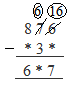Here, we can we see that in the units digit, 6 $-$ * = 7, which means that the value of * is 9, as 1 gets carried from 7 at tens place to 6 at unit place and 6 at unit digit becomes 16   then 16 $-$ 9 = 7.
Now, when 7 gives 1 to 6, it becomes 6, so 6 $-$ 3 = 3.
Also, it can be easily deduced that in ( 8 $-$ * = 6 ), the value of * is 2.

8 7 6
$-$   2 3 9
6 3 7

(ii)

Here, it is clear that in the units place, 9 $-$ 4 = 5; and in the tens place, 8 $-$ 3 = 5.
We can now easily find out the other missing blanks by subtracting 3455 from 8989.

8 9 8 9
$-$   3 4 5 5
5 5 3 4

Addend (difference) = 3455

Thus, the correct answer is :
8 9 8 9
$-$  5 5 3 4
3 4 5 5

(iii)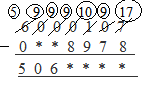Here, in the units digit, 17 $-$ 8 = 9; in the tens digit, 9 $-$ 7 = 2; in the hundreds place, 10 $-$ 9 = 1; and in the thousands place, 9 $-$ 8 = 1.
Addend difference = 5061129
So, in order to get the addend, we will subtract 5061129 from 6000107.
6  0  0  0  1  0  7
$-$  5  0  6  1  1  2  9
0  9  3  8  9  7  8

Thus, the correct answer is:
6  0  0  0  1  0  7
$-$  0  9  3  8  9  7  8
5  0  6  1  1  2  9

(iv)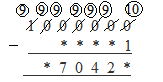In the units place, 10 $-$ 1 = 9. Also, in the lakhs place, 9 $-$ 0 = 9. So, clearly the addend (difference) is 970429.
To find out the other addend, we will subtract 970429 from 1000000.

1  0  0  0  0  0  0
$-$   0  9  7  0  4  2  9
0  0  2  9  5  7  1

Thus, the correct answer is:
1  0  0  0  0  0  0
$-$   0  0  2  9  5  7  1
0  9  7  0  4  2  9

(v)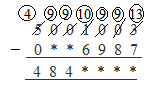Here, in the units digit, 13 $-$ 7 = 6; in the tens digit, 9 $-$ 8 = 1; in the hundreds place, 9 $-$ 9 = 0; and in the thousands place, 10 $-$ 6 = 4.
Addend (difference ) = 4844016
So, to get the other addend, we will subtract 4844016 from 5001003.
5  0  0  1  0  0  3
$-$   4  8  4  4  0  1  6
0  1  5  6  9  8  7

Thus, the other addend is 156987.
The correct answer is:

5  0  0  1  0  0  3
$-$   0  1  5  6  9  8  7
4  8  4  4  0  1  6

(vi)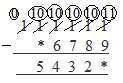It is clear from the units place that 11 $-$ 9 = 2. So, the addend (difference) is 54322.
So, to get the other addend, we will subtract 54322 from 111111.
1  1  1  1  1  1
$-$       5  4  3  2  2
5  6  7  8  9

Thus, the other addend is 56789.
The correct answer is:
1  1  1  1  1  1
$-$       5  6  7  8  9
5  4  3  2  2

#### Question 4:

What is the difference between the largest number of five digits and the smallest number of six digits?

#### Answer:

The largest five-digit number is 99999.
The smallest six-digit number is 100000.
∴ Difference between them = 100000 − 99999 = 1

#### Question 5:

Find the difference between the largest number of 4 digits and the smallest number of 7 digits.

#### Answer:

The largest four-digit number = 9999
The smallest seven-digit number = 1000000
∴ Difference between them = 1000000 − 9999 = 990001

#### Question 6:

Rohit deposited Rs 125000 in his savings bank account. Later he withdrew Rs 35425 from it. How much money was left in his account?

#### Answer:

Money deposited by Rohit  =  Rs 125000
Money withdrawn by Rohit =  Rs 35425
∴ Money left in the account = Rs (125000 − 35425) =  Rs 89575

#### Question 7:

The population of a town is 96209. If the number of men is 29642 and that of women is 29167, determine the number of children.

#### Answer:

Total population of the town  = 96209
Number of men = 29642
Number of women  = 29167
Sum of men and women = (29642 + 29167 ) = 58809
∴ Number of children in the town = (Total population) − (Sum of men and women)
= 96209 − 58809 = 37400

#### Question 8:

The digits of 6 and 9 of the number 36490 are interchanged. Find the difference between the original number and the new number.

#### Answer:

Original number = 39460
New number =   − 36490
Difference =            2970

#### Question 9:

The population of a town was 59000. In one year it was increased by 4536 due to new births. However, 9218 persons died or left the town during the year. What was the population at the end of the year?

#### Answer:

Population of the town = 59000
Increase in the population = 4536
Decrease in the population = 9218
New population = 59000 + 4536 − 9218 = 54318

View NCERT Solutions for all chapters of Class 6